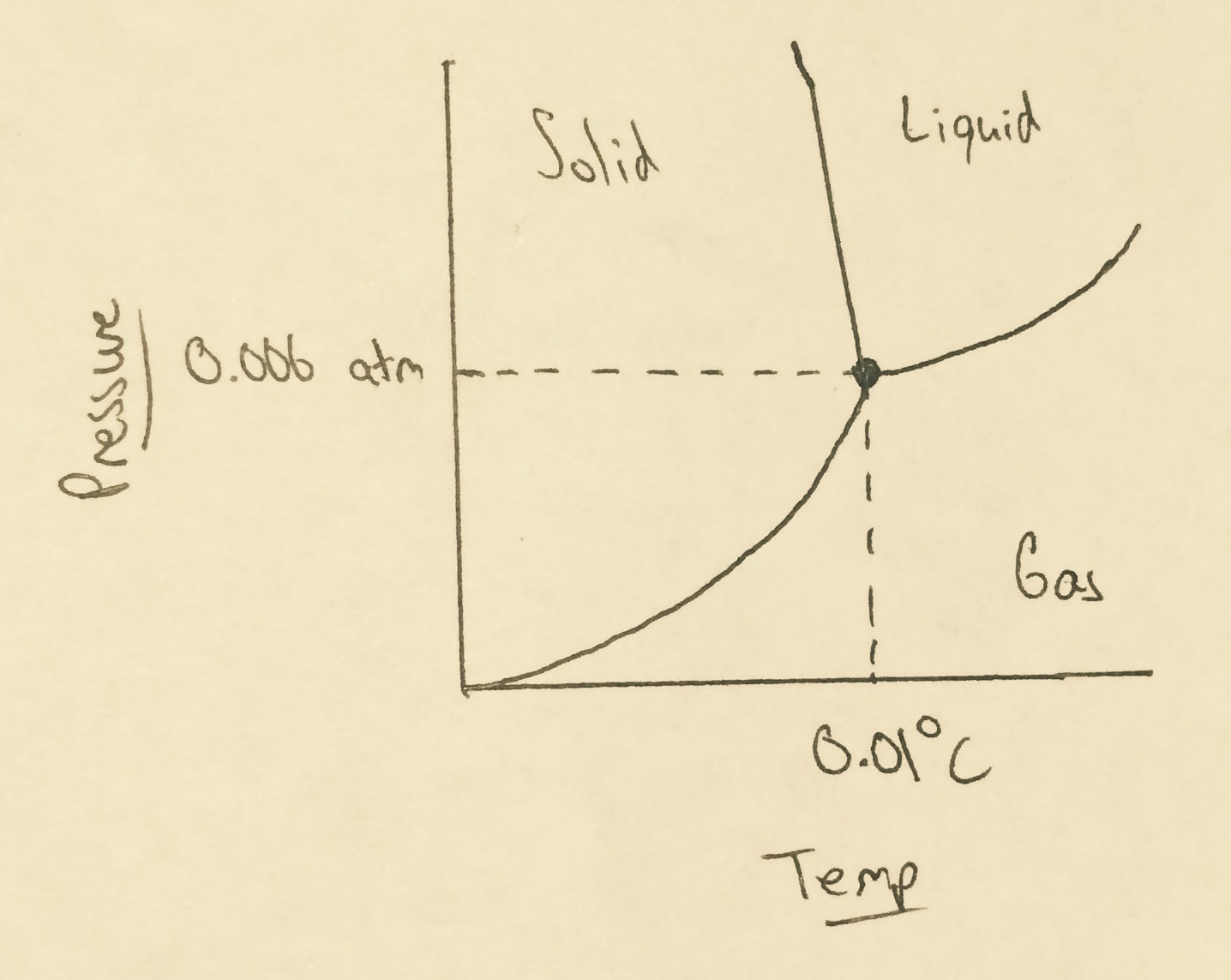# AP Chemistry : Phase Diagrams

## Example Questions

### Example Question #1 : Phase Diagrams

What is the definition of the triple point?

The condensation line of the phase diagram
None of these
The point in which temperature change has no effect on the substance
The state where liquid and gas cannot be differentiated
The state where solid, liquid, and gas coexist in equilibrium
Correct answer: The state where solid, liquid, and gas coexist in equilibrium
Explanation:

Definition of triple point: where solid, liquid, and gas exist in equilibrium

### Example Question #34 : Solutions And States Of Matter

What is represented by point D

Triple point

liquid phase

condensation

evaporation

Critical point

Critical point

Explanation:

the critical point is where the solid and gas cannot be distinguished

### Example Question #2 : Phase Diagrams

A solution of water is at 0.006atm and 0.01 degrees Celsius. What phase(s) are present in the sample?Liquid only

Liquid and gas

Solid and liquid

Solid and gas

Solid, liquid, and gas